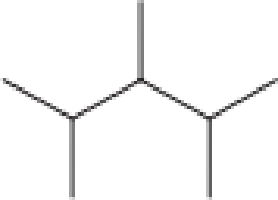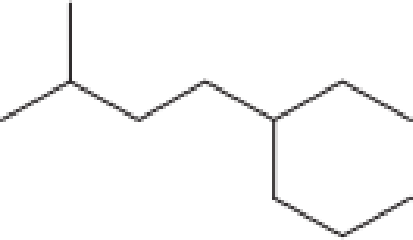Chapter 1, Problem 1.73EP### Organic And Biological Chemistry

7th Edition
STOKER + 1 other
ISBN: 9781305081079

#### Solutions

Chapter
Section### Organic And Biological Chemistry

7th Edition
STOKER + 1 other
ISBN: 9781305081079
Textbook Problem

# Determine the molecular formula for each of the compounds in Problem 12-65.a.b.c.d.(a)

Interpretation Introduction

Interpretation:

Molecular formula for the given compound has to be determined.

Concept Introduction:

Molecular formula is a simple representation of the elements that are present in the compound with the information that how many atoms are present.  From the condensed structural formula the molecular formula can be found.

Molecular formula of the alkanes can be found by using the general formula CnH2n+2.  Here, “n” denotes the number of carbon atoms that is present in the structure.

Explanation

Given line-angle structural formula is,

In line-angle structural representation of molecule, carbon atom is present where two lines meet and at the end of the lines.  In the above structure there are three points where the lines meet and five end points.  Therefore, the total number of carbon atoms present in the given structure is 8.

To determine the molecular formula of alkane, the value of “n” has to be substituted as 8 in the general formula of alkane.

GeneralMolecularFormulaofAlkane = CnH<

(b)

Interpretation Introduction

Interpretation:

Molecular formula for the given compound has to be determined.

Concept Introduction:

Molecular formula is a simple representation of the elements that are present in the compound with the information that how many atoms are present.  From the condensed structural formula the molecular formula can be found.

Molecular formula of the alkanes can be found by using the general formula CnH2n+2.  Here, “n” denotes the number of carbon atoms that is present in the structure.

(c)

Interpretation Introduction

Interpretation:

Molecular formula for the given compound has to be determined.

Concept Introduction:

Molecular formula is a simple representation of the elements that are present in the compound with the information that how many atoms are present.  From the condensed structural formula the molecular formula can be found.

Molecular formula of the alkanes can be found by using the general formula CnH2n+2.  Here, “n” denotes the number of carbon atoms that is present in the structure.

(d)

Interpretation Introduction

Interpretation:

Molecular formula for the given compound has to be determined.

Concept Introduction:

Molecular formula is a simple representation of the elements that are present in the compound with the information that how many atoms are present.  From the condensed structural formula the molecular formula can be found.

Molecular formula of the alkanes can be found by using the general formula CnH2n+2.  Here, “n” denotes the number of carbon atoms that is present in the structure.

### Still sussing out bartleby?

Check out a sample textbook solution.

See a sample solution

#### The Solution to Your Study Problems

Bartleby provides explanations to thousands of textbook problems written by our experts, many with advanced degrees!

Get Started